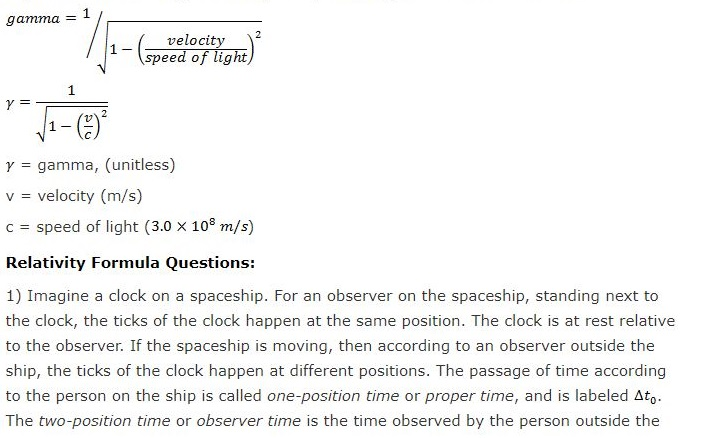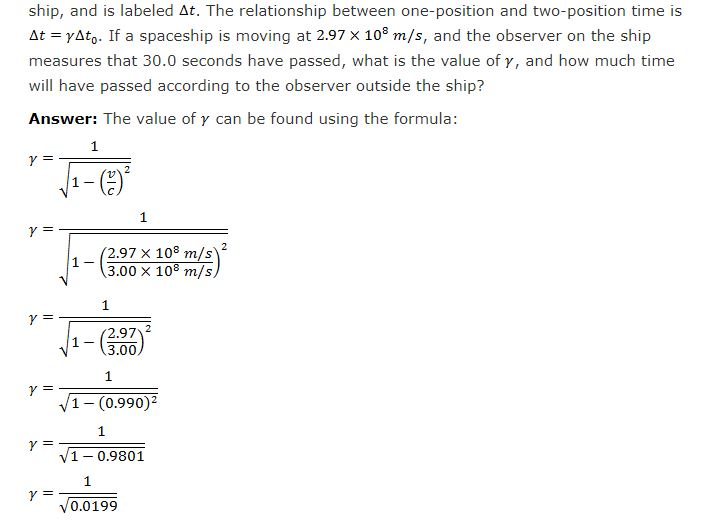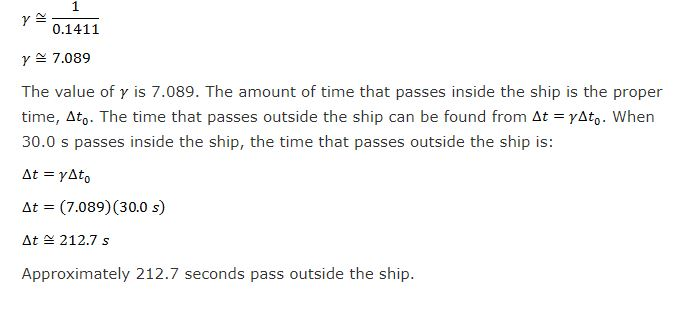# Relativity Formula

As per the theory of Special relativity, length, time, momentum, and energy depends on the velocity of one reference frame relative to another. A person on a spaceship moving near the light speed will measure length, time, momentum, and energy differently than an observer that is outside the ship. The formula that relates a value in one reference frame to the value in another is labelled with the Greek letter  (“gamma”). It is unitless and depends on the velocity divided by the light speed.More topics in Relativity Formula Time Dilation Formula Length Contraction Formula Relative Velocity Formula